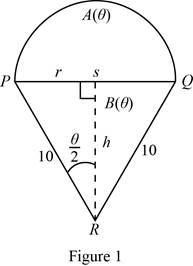# The value of lim θ → 0 + A ( θ ) B ( θ ) .### Single Variable Calculus: Concepts...

4th Edition
James Stewart
Publisher: Cengage Learning
ISBN: 9781337687805### Single Variable Calculus: Concepts...

4th Edition
James Stewart
Publisher: Cengage Learning
ISBN: 9781337687805

#### Solutions

Chapter 3.3, Problem 48E
To determine

## To find: The value of limθ→0+A(θ)B(θ) .

Expert Solution

The value of limθ0+A(θ)B(θ)=0.

### Explanation of Solution

Given:

The area of the semicircle is A(θ) and the area of the triangle is B(θ).

Formula used:

The area of the semicircle is 12πr2 (1)

The area of the triangle is 12bh (2)

Calculation:

The given picture is shown in the below Figure 1.From Figure 1, the base of the triangle (PQ) is same as the diameter of semicircle (2r).

Calculate r and h.

From the triangle PSR,

sinθ2=opposite sidehypotenuse=r10r=10sinθ2

And

Substitute b=2r in equation (2),

B(θ)=12(2r)h=rh

Thus, the area of the triangle is B(θ)=rh.

From equation (1), the area if the semicircle is A(θ)=12πr2.

Consider limθ0+A(θ)B(θ).

Substitute A(θ)=12πr2 and B(θ)=rh,

limθ0+A(θ)B(θ)=limθ0+12πr2rh=limθ0+12πrh=π2limθ0+rh

Substitute r=10sinθ2 and h=10cosθ2,

limθ0+A(θ)B(θ)=π2limθ0+10sinθ210cosθ2=π2limθ0+(tanθ2 )    (Qsinxcosx=tanx)=π2tan(0)=0

Therefore, the value of limθ0+A(θ)B(θ)=0.

### Have a homework question?

Subscribe to bartleby learn! Ask subject matter experts 30 homework questions each month. Plus, you’ll have access to millions of step-by-step textbook answers!